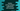# Python string hexdigits explanation with example## Python string hexdigits explanation with example:

Python string hexdigits is a string constant that contains all hexadecimal characters. It includes all these characters: ‘0, 1, 2, 3, 4, 5, 6, 7, 8, 9, a, b, c, d, e, f, A, B, C, D, E, F’.

This is a constant defined in python string. To access this value, we need to import string library by using import string. We can then access this constant: string.hexdigits.

## Definition:

``string.hexdigits``

It returns a string that includes all hexadecimal characters.

## Example program:

Let’s take a look at the below program:

``````import string

print(string.hexdigits)``````

If you run this program, it will print the below output:

``0123456789abcdefABCDEF``

As you can see here, it prints all hexadecimal characters in a string.

### Python program to check if a string has only hexadecimal characters:

Let’s write a program to check if all characters in a string includes all hexadecimal characters. We can use string.hexdigits for that. Below is the complete program:

``````import string

def check_hexa(given_str):
for ch in given_str:
if ch not in string.hexdigits:
return False
return True

input_str = input('Enter a string: ')

if(check_hexa(input_str)):